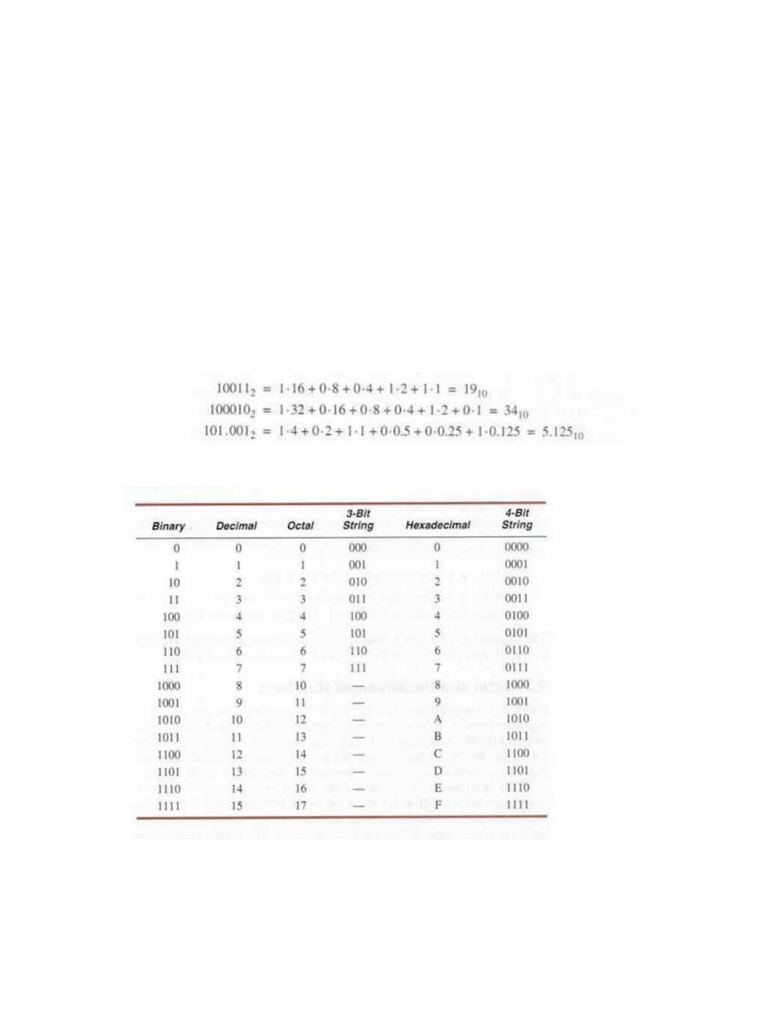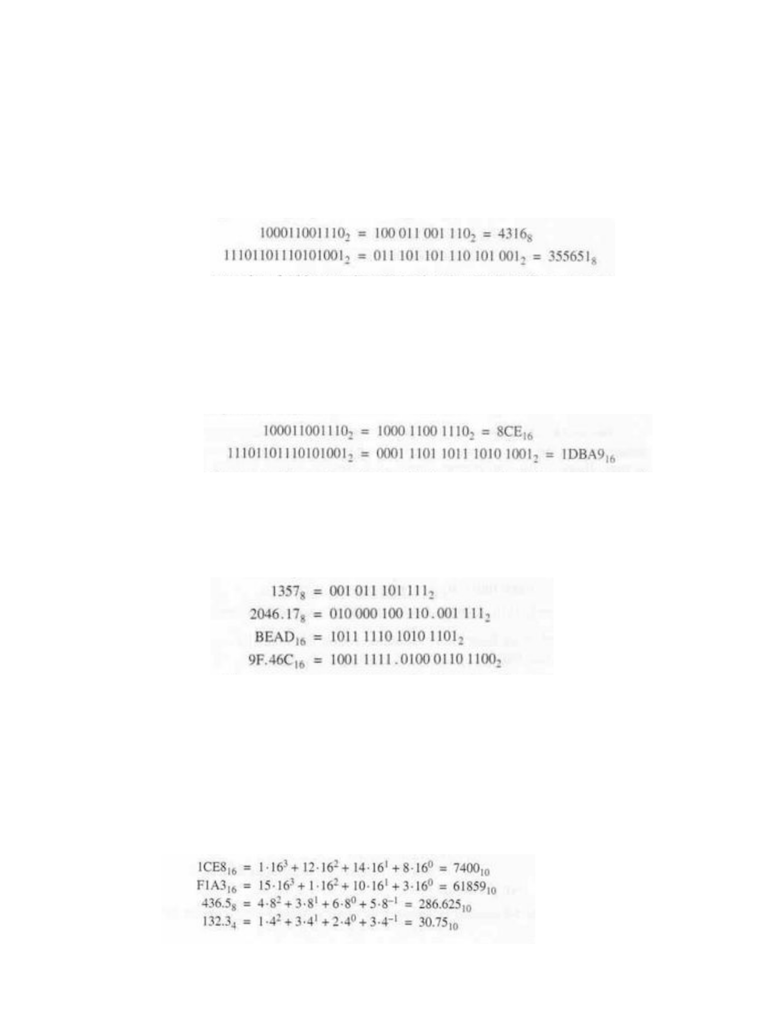# 14:332:231 Lecture Notes - Lecture 2: Radix, Octal, Negative Number

41 views7 pagesCHAPTER TWO: NUMBER SYSTEMS AND CODES
2.1 Positional Number System
Positional Number System: number represented by a string of digits
Each digit position has an associated weight
1734 = 1*1000 + 7*100 + 3*10 + 4*1
Here, 10 is called the base
is where there are p digits to the left and n digits to the right of
a point
Most Significant Digit (MSD): leftmost digit
Least Significant Digit (LSD): rightmost digit
Binary Digits (Bits): used with values 0 and 1 to represent digital signals, has binary
Examples:
Octal: radix r=8 (needs 8 digits), uses digits 0-7
Conversions
Unlock document

This preview shows pages 1-2 of the document.
Unlock all 7 pages and 3 million more documents.Binary-to-Octal
Separate bits into groups of 3
Start separating from the right and fill in zeros to the left to create the
groups of 3
Replace each set of 3 with the corresponding octal digit
Example:
Separate bits into groups of 4
Start separating from the right and fill in zeros to the left to create the
groups of 4
Replace each set of 4 with the corresponding hexadecimal digit
Example:
Replace each octal or hexadecimal digit with the corresponding 3 or 4 bit
string that is binary
Examples:
0x Prefix: prefix used to denote a hexadecimal number (Ex: 0xBFC0000)
2.3 General Positional-Number-System Conversions
Simply evaluate
Take each digit of radix r and define it based on the radix
Examples:
Unlock document

This preview shows pages 1-2 of the document.
Unlock all 7 pages and 3 million more documents.